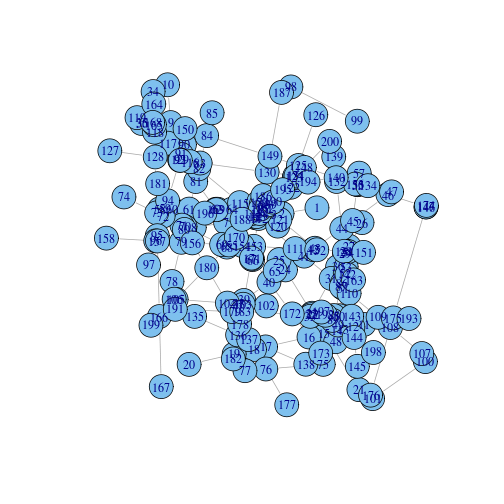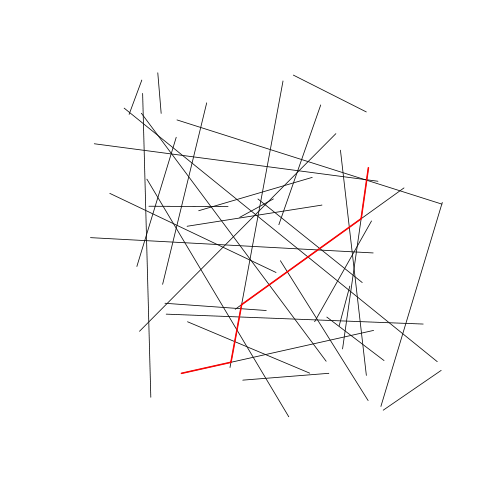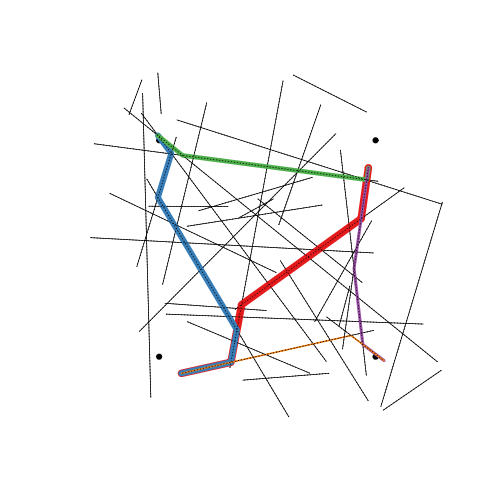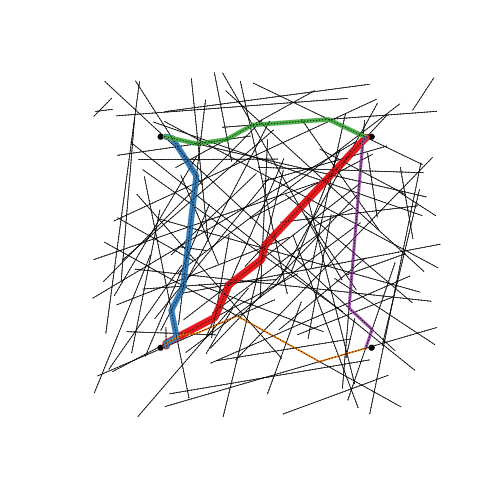# Line to Graph to Route

## Introduction

This document shows how to go from a set of lines to an `igraph` network object, and then do shortest path routing on that network.

## Requirements

``````require(igraph)
require(RColorBrewer)
require(sp)
require(ANN)
require(rgeos)
``````

## Make Some Data

First a function to generate some sample data. This code generates a number of random segments on the unit square, and converts them to a `SpatialLines` object. I think of this as like a nest of twigs.

``````makeNest <- function(n) {
### generate a bunch of SpatialLines on (0,1) square
xy = cbind(runif(n), runif(n), runif(n), runif(n))
lines = Lines(apply(xy, 1, function(z) {
Line(rbind(z[1:2], z[3:4]))
}), ID = 1)
SpatialLines(list(lines))
}

gg = makeNest(35)
plot(gg)
``````## Build Topology

This function builds the `igraph` object. The fundamental trick is to intersect the lines with themselves. This creates vertices at all the end points and intersections. The graph is built with Euclidean distance as edge weight, and with the x and y coordinates attributed to the vertices. The plot method uses the x and y coordinates for the layout, but the large vertex symbols obscure things a bit.

``````buildTopo <- function(lines) {

g = gIntersection(lines, lines)
edges = do.call(rbind, lapply(g@lines[]@Lines, function(ls) {
as.vector(t(ls@coords))
}))
lengths = sqrt((edges[, 1] - edges[, 3])^2 + (edges[, 2] - edges[, 4])^2)

froms = paste(edges[, 1], edges[, 2])
tos = paste(edges[, 3], edges[, 4])

graph = graph.edgelist(cbind(froms, tos), directed = FALSE)
E(graph)\$weight = lengths

xy = do.call(rbind, strsplit(V(graph)\$name, " "))

V(graph)\$x = as.numeric(xy[, 1])
V(graph)\$y = as.numeric(xy[, 2])
return(graph)
}

graph = buildTopo(gg)
plot(graph)
``````## Shortest Path Routing

This function maps two points (as one-row, two-column matrices) to the nearest node on the network then computes the vertices of the shortest path. We compute a route roughly across the diagonal of the unit square.

``````routePoints <- function(graph, from, to) {
require(FNN)
xyg = cbind(V(graph)\$x, V(graph)\$y)

ifrom = get.knnx(xyg, from, 1)\$nn.index[1, 1]
ito = get.knnx(xyg, to, 1)\$nn.index[1, 1]

p = get.shortest.paths(graph, ifrom, ito, output = "vpath")
p[]
}

route1 = routePoints(graph, cbind(0.2, 0.2), cbind(0.8, 0.8))
``````
``````## Loading required package: FNN
``````

## Plotting Routes

We can plot routes simply by getting the x and y coordinates of the vertices.

``````plotRoute <- function(graph, nodes, ...) {
lines(V(graph)[nodes]\$x, V(graph)[nodes]\$y, ...)
}

plot(gg)
plotRoute(graph, route1, lwd = 2, col = "red")
``````## Further Examples

This code computes four more routes parallel to the sides of the unit square. We add the origin points to the plot and finally overplot the lines again to show that the paths really follow the network.

``````route2 = routePoints(graph, cbind(0.2, 0.2), cbind(0.2, 0.8))
route3 = routePoints(graph, cbind(0.2, 0.8), cbind(0.8, 0.8))
route4 = routePoints(graph, cbind(0.8, 0.8), cbind(0.8, 0.2))
route5 = routePoints(graph, cbind(0.8, 0.2), cbind(0.2, 0.2))
colours = brewer.pal(5, "Set1")
plot(gg)
points(rbind(c(0.2, 0.2), c(0.2, 0.8), c(0.8, 0.8), c(0.8, 0.2)),
pch = 19)
plotRoute(graph, route1, lwd = 10, col = colours)
plotRoute(graph, route2, lwd = 8, col = colours)
plotRoute(graph, route3, lwd = 6, col = colours)
plotRoute(graph, route4, lwd = 4, col = colours)
plotRoute(graph, route5, lwd = 2, col = colours)
plot(gg, add = TRUE, lty = 3)
``````Adding more points to the graph produces a more complex network and the routes more closely approximate the sides and diagonal of the square.

``````gg = makeNest(100)
graph = buildTopo(gg)
route1 = routePoints(graph, cbind(0.2, 0.2), cbind(0.8, 0.8))
route2 = routePoints(graph, cbind(0.2, 0.2), cbind(0.2, 0.8))
route3 = routePoints(graph, cbind(0.2, 0.8), cbind(0.8, 0.8))
route4 = routePoints(graph, cbind(0.8, 0.8), cbind(0.8, 0.2))
route5 = routePoints(graph, cbind(0.8, 0.2), cbind(0.2, 0.2))
colours = brewer.pal(5, "Set1")
plot(gg)
points(rbind(c(0.2, 0.2), c(0.2, 0.8), c(0.8, 0.8), c(0.8, 0.2)),
pch = 19)
plotRoute(graph, route1, lwd = 10, col = colours)
plotRoute(graph, route2, lwd = 8, col = colours)
plotRoute(graph, route3, lwd = 6, col = colours)
plotRoute(graph, route4, lwd = 4, col = colours)
plotRoute(graph, route5, lwd = 2, col = colours)
plot(gg, add = TRUE, lty = 3)
``````## Notes

If the finish point can't be reached from the start point then `plotRoute` will stop with an error.

Only one shortest path is returned, even if there are multiple equally-short paths.